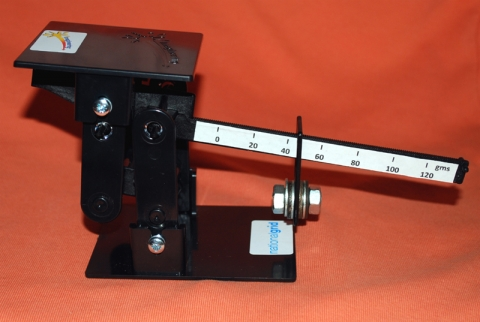# Letter Balance

Junior Engineering

Weighing machines either compare the weight of the object against a standard or measure the gravitational force the object exerts on the machine. A spring balance is an example of the latter. Electronic balances are just ‘electrical’ spring balances with an electronic display. This Letter Balance is of the comparative type where the turning force of each arm of the comparative balance
exerts a bending force or moment on the balance beam and is in equilibrium when these are equal. The bending moment is the product of the force and distance to the pivot point. In a conventional balance the distances between the pivots to the points where the force or weight acts, are equal. This balance is known as a Steelyard Balance where the moment on one side of the pivot is adjusted to be equal to that of the other.## Key Points

• Will weigh objects such as a letter upto 120 grams
• Uses screwdriver, spanners
• Duration 3-4 weeks

## Topics covered

• Bending moments
• Equilibrum of moments
• Mathematical equations
Back to project library